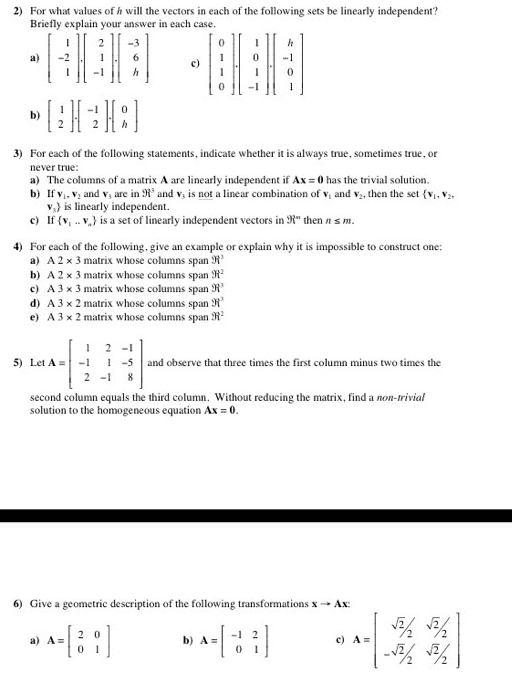2
0
watching
134
views10 Nov 2019

# please exaplin these clearly.For what values of h will the vectors in each of the following sets he linearly independent? Briefly explain your answer in each case. [1 -2 1],[2 1 -1],[-3 6 h] [1 2],[-1 2],[0 h] [0 1 1 0],[1 0 1 -1],[h -1 0 1] For each of the following statements, indicate whether it is always true, sometimes true, or never true: The columns of a matrix A are linearly independent if Ax = 0 has the trivial solution. If v1, v2 and v3 are in R3 and v3 is not a linear combination of v1 and v2, then the set {v1, v2, v3} is linearly independent. If {v1 .. v } is a set of linearly independent vectors in R then n m. For each of the following, give an example or explain why it is impossible to construct one: A 2 times 3 matrix whose columns span R3 A 2 times 3 matrix whose columns span R2 A 3 times 3 matrix whose columns span R3 A 3 times 2 matrix whose columns span R3 A 3 times 2 matrix whose columns span R2 Let A = [1 2 -1 -1 1 -5 2 -1 8] and observe that three times the first column minus two tunes the second column equals the third column. Without reducing the matrix, find a non-trivial solution to the homogeneous equation Ax = 0. Give a geometric description of the following transformations x rightarrow Ax: A = [2 0 0 1] A = [-1 2 0 1] A = [ /2 /2 /2 /2]